## Project 4 / Scene Recognition with Bag of Words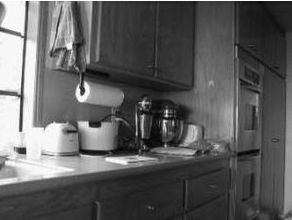->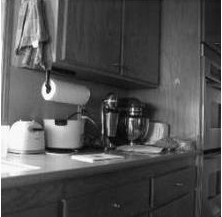Cropping a training image without changing its aspect ratio.

Our goal for this project is to perform scene recognition with a variety of feature quantization and image representation techniques. The two primary methods employed in the project are tiny image representation and the use of a Bag of visual words model. It is worth noting that a number of extensions to the image representation and classification model such as the use of fisher encoding and training with RBF kernels and soft assignment have been attempted to improve the robustness of my scene regonition algorithm.

The general pipeline of large instance scene recognition consists of two categories of image representation and two methods of classification overall. The simplest mechanism which I implemented first is the combination of tiny image representation and nearest neighbor classifier.

#### Combinations of recognition pipeline implemented:

• Tiny images representation + vanilla classifier
• Tiny images representation + Nearest neighbor classifier
• Bag of SIFT + linear SVM classifier
• Bag of SIFT + SVM classifier with RBF kernel
• Bag of SIFT + SVM classifier + Soft Assignment
• Fisher Encoded SIFT + SVM classifier

For purposes of verification we test our image recognition algorithm on a database of 15-category images widely used for benchmarking purposes. Each category is represented by 100 images giving a total of 1500 images for our algorithm to "learn" its classification model from. Initially, we showed that compared to the baseline measure, just downsizing the image to a 16x16 framesize along with a nearest neighbor classifier simply gives a naive yet modest accuracy improvement of about 12-15%.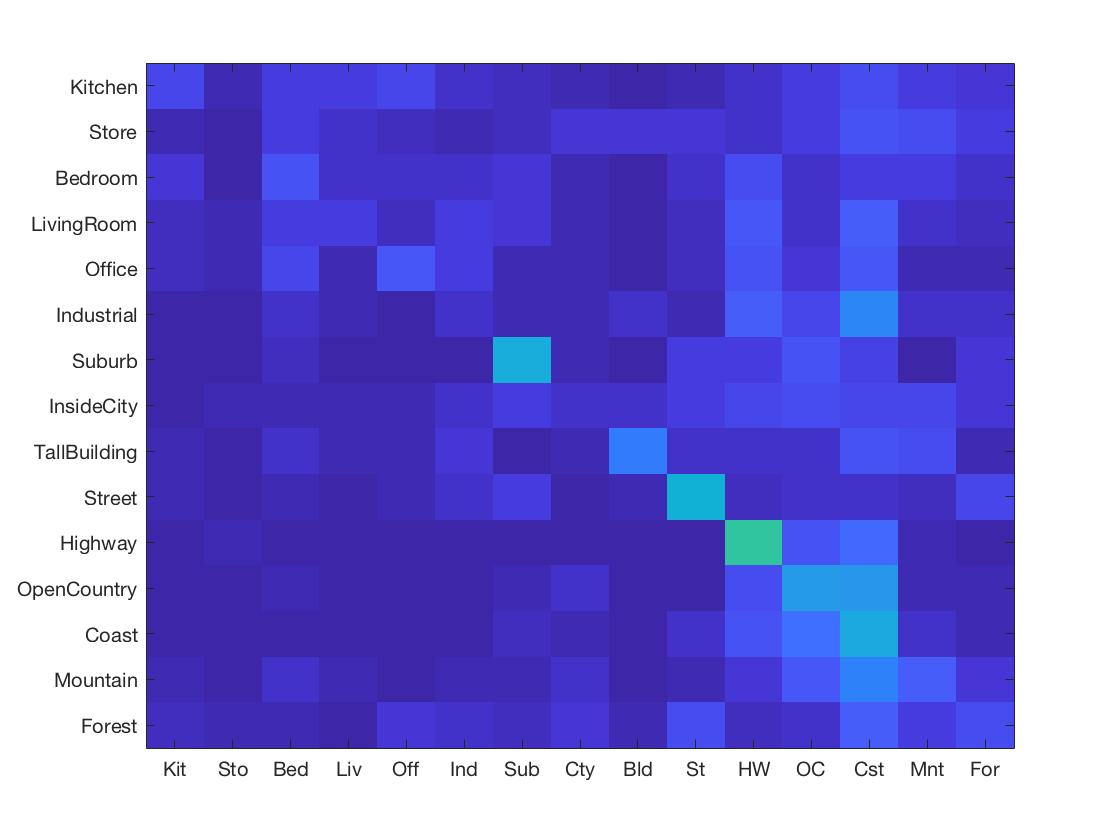Despite its simplicity, nearest neightbor classification can be very useful even with only one neighbor computed (in the case there're > 1 neighbor computed, as required in 'soft assignment', it inself is a k-nearest-neighbors subroutine). Combined with the Bag of visual words model, I was able to increase its accuracy to about ~55%. To achieve this level of accuracy, a good amount of finetuning of the parameters was required as I chose to stick with the optimizing set of vocab size = 400, step size (vocab) = 10, step size (BoW) = 5 and lambda = 0.01 in the end for best performance. To implement nearest neighbors, I preprocessed a matrix of edge distances between train vs. test points in feature space using VLFEAT's`vl_alldist2` and iterated through every image assigning it the label of its closest neighbor. As for the bag of visual words implementation, I sampled the image for SIFT features with a fine-tuned step size first and then clustered them into a specified cluster size using the kmeans algorithm, as shown below.

### Bag of Words + Nearest Neighbor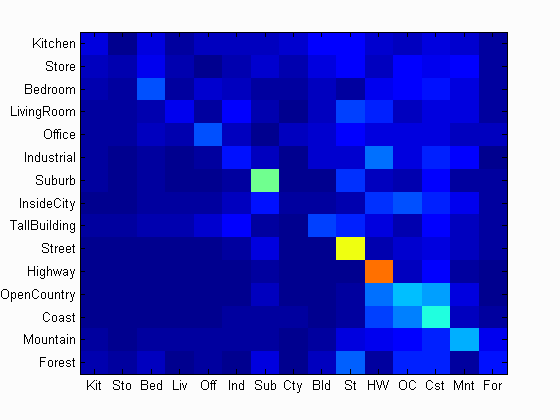``````
% Nearest Neighbor Algorithm
predicted_categories = [];
numImages = size(test_image_feats, 1);

train_pts = train_image_feats';
test_pts = test_image_feats';
dist = vl_alldist2(train_pts, test_pts);
[mdist, idx] = min(dist);

for i=1:numImages
nearest_idx = idx(i);
predicted_categories = [predicted_categories; train_labels(nearest_idx)];
end
``````

up from 22.9% accuracy to 55% with Bag of Words model introduced

``````
% Basic Bag of Words Algorithm
numImages = size(image_paths,1);
stepSize = 10;
samp_feats = [];

for i=1:numImages
[locations, SIFT_features] = vl_dsift(single(img), 'step', stepSize, 'fast');
samp_feats = [samp_feats SIFT_features];
end
[centers, assignments] = vl_kmeans(single(samp_feats), vocab_size);
vocab = centers;
``````

So how do we do better? Humans generally have at least 60% recognition accuracy and we would like for machines to approach that range too. One way is to have the machines learn a better representation of the image. Instead of using the nearest neighbor classifier which is rather efficient in complexity while accounting to a good degree for the image's structure, I'd implemented a linear support vector machine (SVM) that does binary classification on new images given a dataset as input to pre-train its model on. And surprisingly despite being a binary classifier that is more lossy compared to the nearest neighbor classifier, it worked very well as it was able to learn what underlying structures are important and throw away those that are less relevant. Using the bag of words and linear SVM pipeline I was able to get 63.6% accuracy, which is well over 50%.

### Extra Credit

To further improve the performance of my scene recognition pipeline, I've tried to extend and tweak a couple implementation subroutines and parameters as listed below. First I experimented with many different vocabulary sizes for our Bag of words unsupervised clustering model, with the following improvements made:

#### vocab size = 10, 20, 50, 100, 200, 400 (left to right)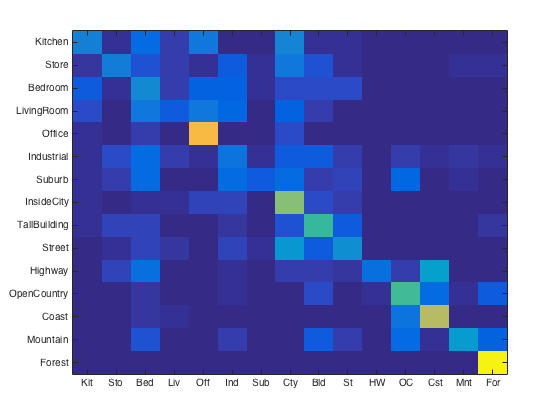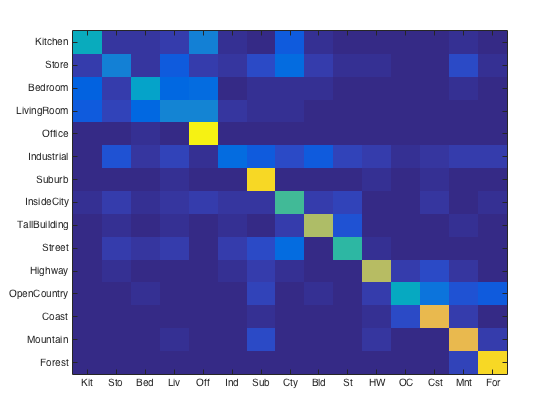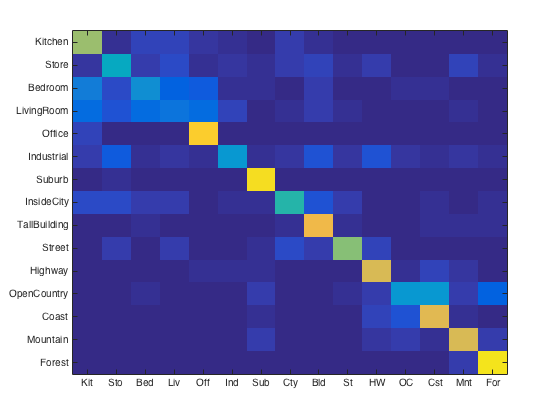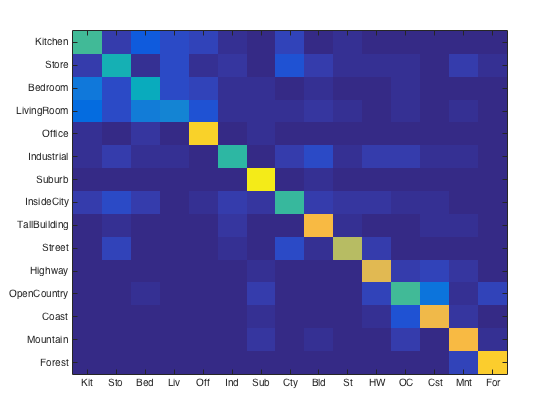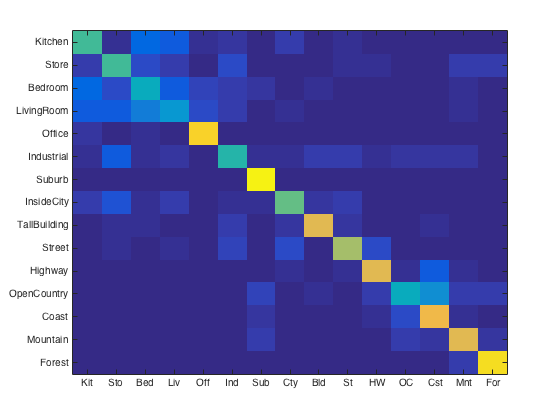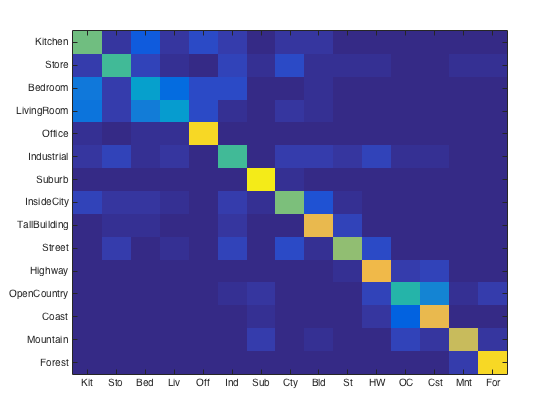#### lambda = 0.0001 --> 10, with step multiplier = 10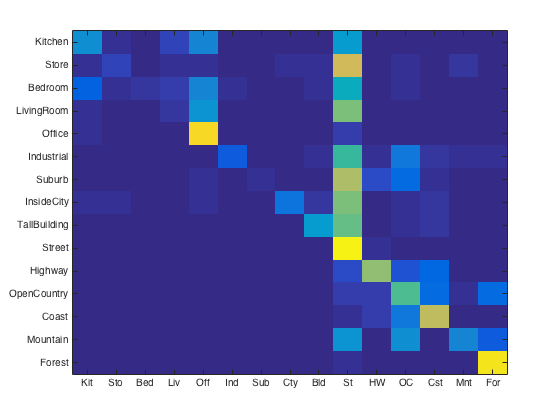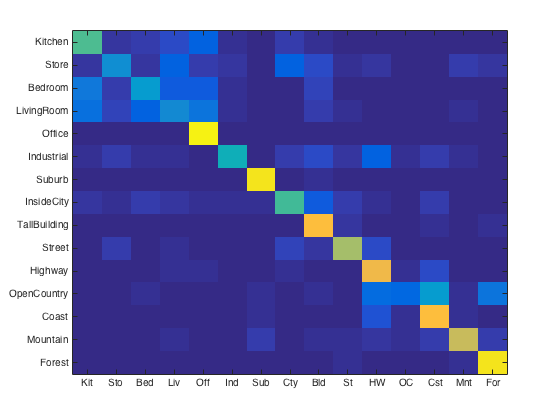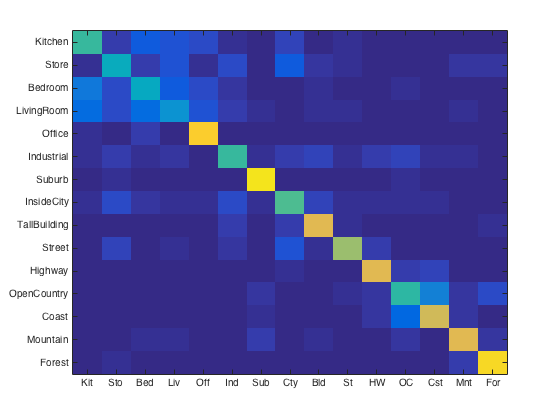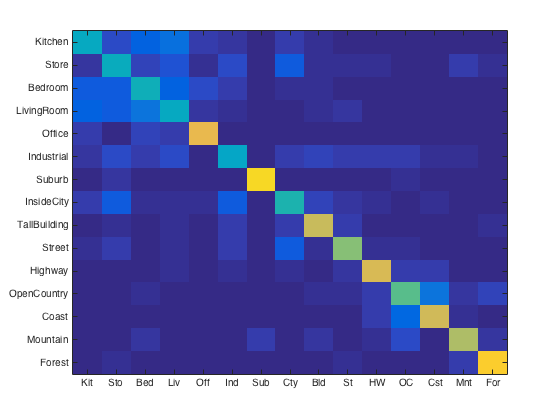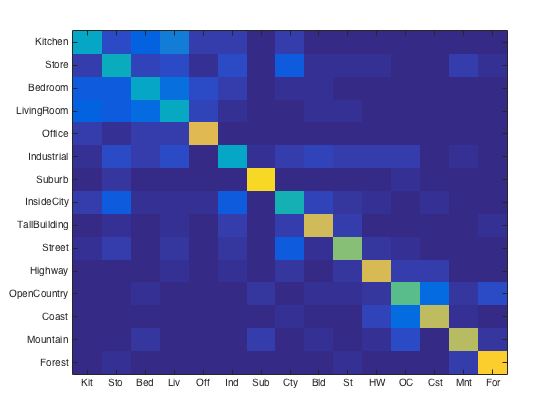#### Soft Assignment in `get_bags_of_sifts.m`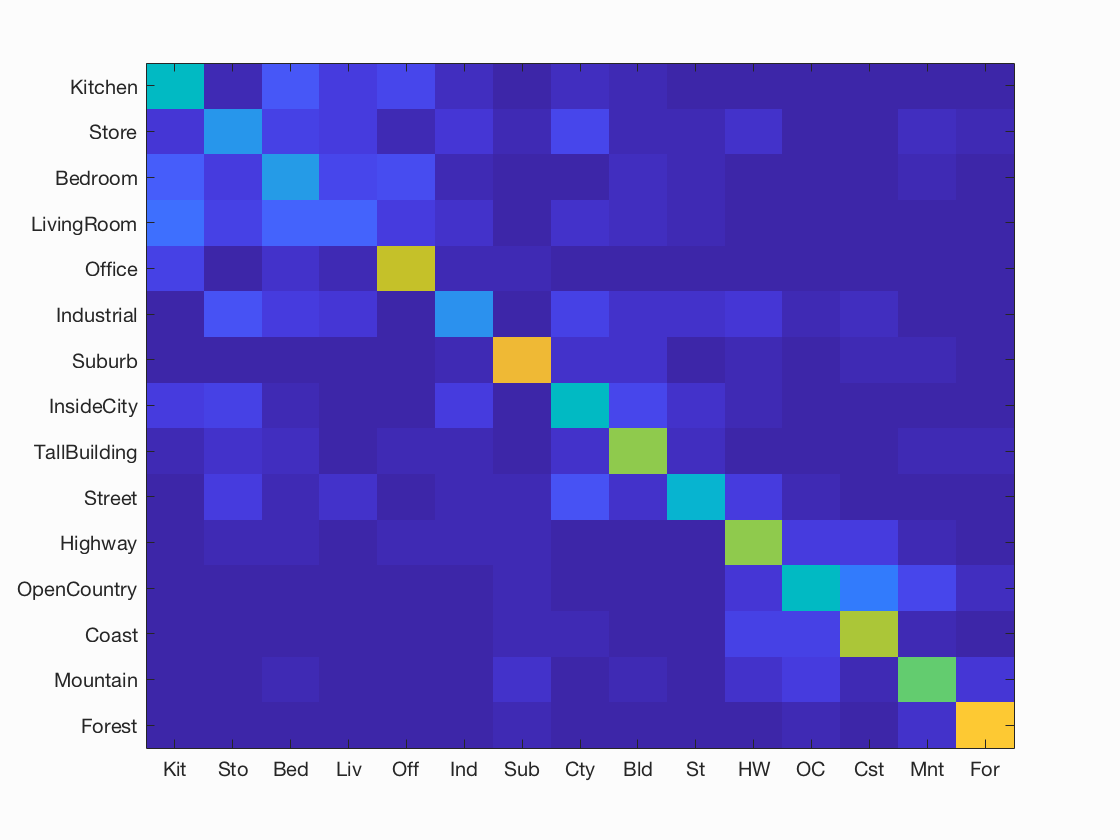Instead of matching correspondences to the closest bin, which ignores the fact that points in feature space can be very close to each other in reality but infinitely divided due to the locality of cluster centoids, I'd instead ran an underlying k-nearest-neighbor algorithm to allocate different weights to each histogram bin depending on the distance an image feature is from its nearby centoids.

#### Training SVM on RBF kernel with Olivier Chapelle's SVM code

``````
% Using rbf code found online, original author: Tijl De Bie
n=size(coord,1);
K=coord*coord'/sig^2;
d=diag(K);
K=K-ones(n,1)*d'/2;
K=K-d*ones(1,n)/2;
K=exp(K);

% main code in svm_classify_kern.m
% written to support other kernels as well.
``````

As a surprise, performance dropped to about ~57%. Due to the lengthy time it takes to retrain and reconstruct visual vocabularies, I wasn't able to experiment with smaller step sizes (i.e. < 5) as MATLAB would become unresponsive after running the program for a while (had to force quit after a run for step size = 3 after 2 hours 6 mins).

#### Fisher Encoding of feature vectors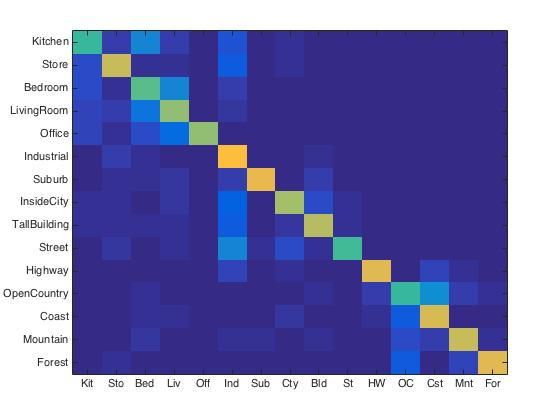Code style modeled after `build_vocabulary.m` and `get_bags_of_sifts.m`. Builds a vector of Nxd means, covariances and priors using VLFEAT's `vl_gmm()` for its gausian mixture model then saving them to avoid recomputation on every run.

``````
%% get_fisher_encoding.m %%

numImages = size(image_paths, 1);
stepSize = 10;
image_feats = [];

for i=1:numImages